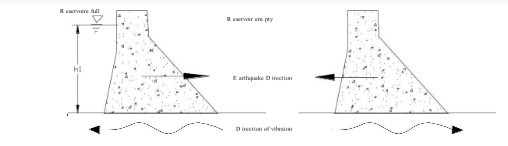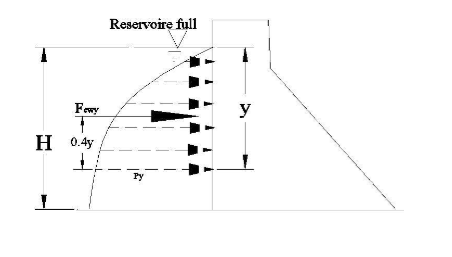### Earthquake Forces

Earthquake Forces

• Dynamic loads generated by seismic disturbances must be considered in the design of all major dams situated in recognized seismic “high risk” regions. The possibility of seismic activity should also be considered for dams located outside those regions, particularly sites in close proximity to potentially active geological fault complexes.

• Seismic activity is associated with complex oscillating patterns of accelerations and ground motions, which generate transient dynamic loads due to the inertia of the dam and the retained body of water. For design purposes both should be considered operative in the sense least favorable to stability of the dam. Horizontal accelerations are therefore assumed to operate normally to the axis of the dam. Under reservoir full conditions the most adverse seismic loading will then occur when a ground shock is associated with:

1. Horizontal foundation acceleration operating upstream, and

2. Vertical foundation acceleration operating downward• Direction of ground acceleration and the respective horizontal earthquake force on gravity dam

• As a result of 1, inertia effects will generate an additional hydrodynamic water load acting downstream, plus a further inertia load attributable to the mass of the dam and also acting in a downstream sense. Foundation acceleration downwards, 2 above, will effectively reduce the mass of the structure. The more important recurring seismic shock waves have a frequency in the range 1-10Hz. Seismic loads consequently oscillate very rapidly and are transient in their effect. The strength of seismic event can be characterized by its magnitude and its intensity.

• Ground motions associated with earthquakes can be characterized in terms of acceleration, velocity or displacement. Only peak ground acceleration, pga, generally expresses as a portion of gravitational acceleration, g, is considered in this course. It has been suggested that in general seismic events with a high pga of short duration are less destructive than seismic events of lower pga and greater duration.

• The natural frequency of vibration, fn, for a triangular gravity profile of height H (m) and base thickness B(m) constructed in concrete with an effective modulus of elasticity E=14GPa can be
approximated as fn = 600 B/H2 (Hz)

• For a dam of H = 500m and B = 375m, fn = 0.9 Hz. But the most important recurring seismic shock waves are in the order of magnitude of 1-10Hz. Hence resonance (the frequency of vibration of the structure and earthquake are equal) of an entire dam is unlikely and is not a series concern in design. But vulnerable portion of the dam should be detailed.

There are two methods to determine the seismic load on a dam:

• Pseudostaic (equivalent static load) method: inertia forces are calculated based on the acceleration maxima selected for design and considered as equivalent to additional static loads.  This method generally is conservative and is applied to small and less vulnerable dams.

• The acceleration intensities are expressed by acceleration coefficients h (Horizontal) and

• v(vertical) each representing the ratio of peak ground acceleration. Horizontal and vertical  accelerations are not equal, the former being of greater intensity (h = (1.5 – 2.0v).

• Based on the vertical and horizontal acceleration, the inertial force will be
Horizontal force = ±h * (static mass)
Vertical force  = ±v * (static mass)

• Peak horizontal ground acceleration with zero vertical ground acceleration

• Peak vertical ground acceleration with zero horizontal acceleration

• Appropriate combination of both (eg. Peak of the horizontal and 40-50% of the vertical)Inertia Forces

1. Mass of dam
Horizontal  Feqh = ±hW
Vertical  Feqv = ±vW

2. Water body
As analyzed by Westerguard(1993)
Py k ' ' h
w H. y
2  y   H . y k '
F  ewy     h  w  '
3

where k” = earthquake factor for the water body

k "  0 .816

Where: T = period of earthquake

3

w = in tone/m H, y in meters

The force acts at 0.4y from the dam joint being considered. For inclined upstream face of dam

H . y

Py    k ' ' h w  cos b

where b is the angle the face makes with the vertical.

The resultant vertical hydrodynamic load, Fewv, effective above an upstream face batter or flare may be accounted for by application of the appropriate seismic coefficient to vertical water load.  It is considered to act through the centroid of the area.

Fewv = ±v Fv

Uplift load is normally assumed to be unaltered by seismic shock.

Dynamic analysis:
The dam is idealized as a two dimensional plane-strain or plane-stress finite element system, the reservoir being regarded as a continuum. The foundation zone is generally idealized as a finite element system equivalent to a visco-elastic half space. The complexities of such an approach are evident, and take it outside the scope of this course.